# 机器学习基础：用 Lasso 做特征选择

Lasso 给简单线性回归加了 L1 正则化，可以将不重要变量的系数收缩到 0 ，从而实现了特征选择。本文重点也是在讲解其原理后演示如何用其进行特征选择，希望大家能收获一点新知识。

## lasso 原理

Lasso就是在简单线性回归的目标函数后面加了一个1-范数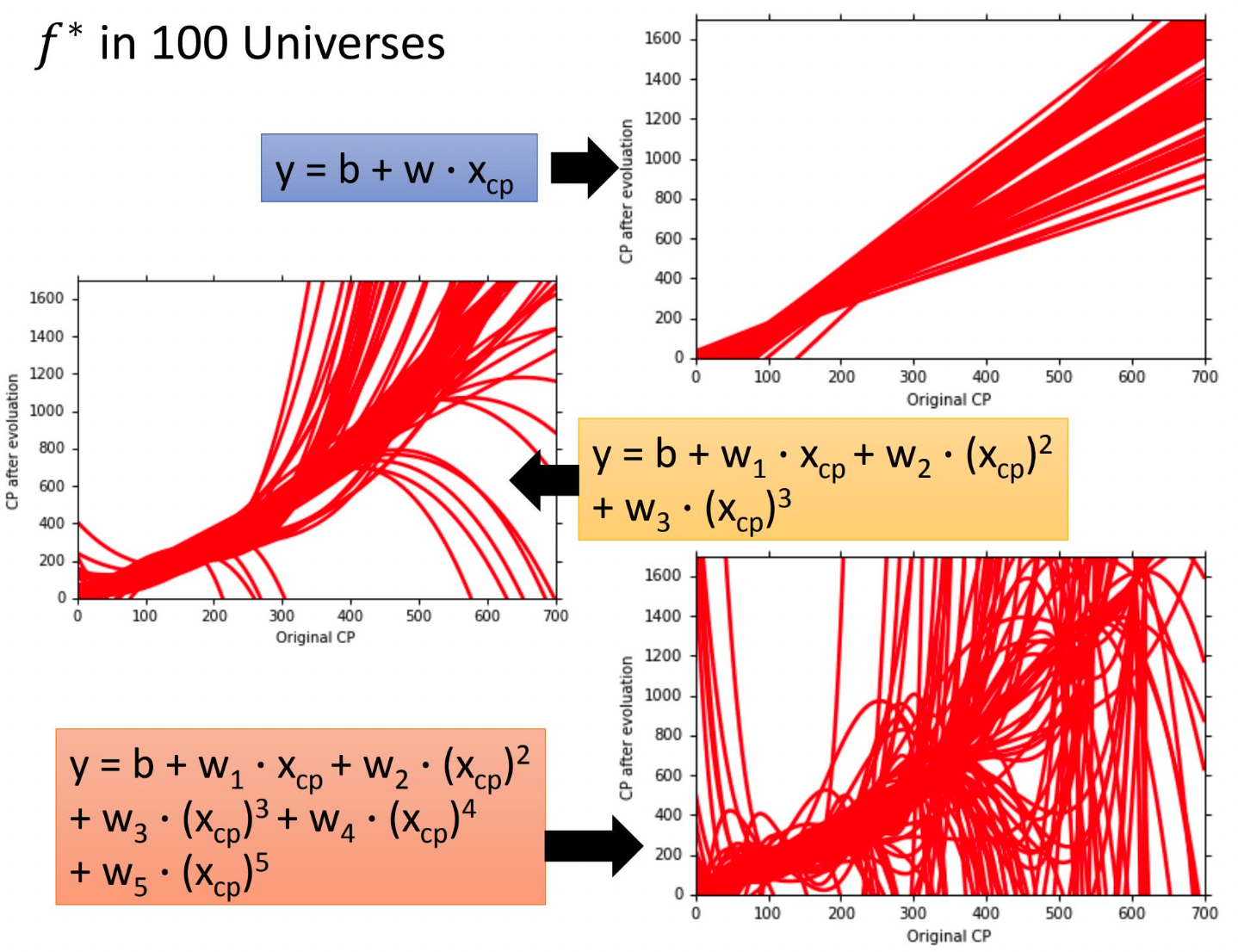lasso回归的代价函数 $$J(\theta)=\frac{1}{2}\sum_{i}^{m}(y^{(i)}-\theta ^Tx^{(i)})^2+\lambda \sum_{j}^{n}|\theta_j|$$ 矩阵形式： $$J(\mathbf\theta) = \frac{1}{2n}(\mathbf{X\theta} - \mathbf{Y})^T(\mathbf{X\theta} - \mathbf{Y}) + \alpha||\theta||_1$$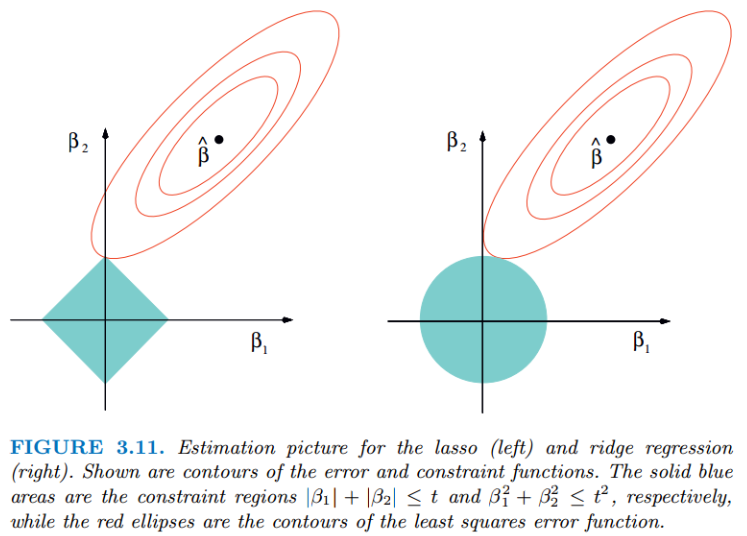https://www.cnblogs.com/pinard/p/6018889.html

https://arxiv.org/pdf/math/0406456.pdf

scikit-learn 提供了这两种优化算法的Lasso实现，分别是  sklearn.linear_model.Lasso(alpha=1.0, *, fit_intercept=True, normalize='deprecated', precompute=False, copy_X=True, max_iter=1000, tol=0.0001, warm_start=False, positive=False, random_state=None, selection='cyclic')

sklearn.linear_model.lars_path(X, y, Xy=None, *, Gram=None, max_iter=500, alpha_min=0, method='lar', copy_X=True, eps=2.220446049250313e-16, copy_Gram=True, verbose=0, return_path=True, return_n_iter=False, positive=False) 

## 用 Lasso 找到特征重要性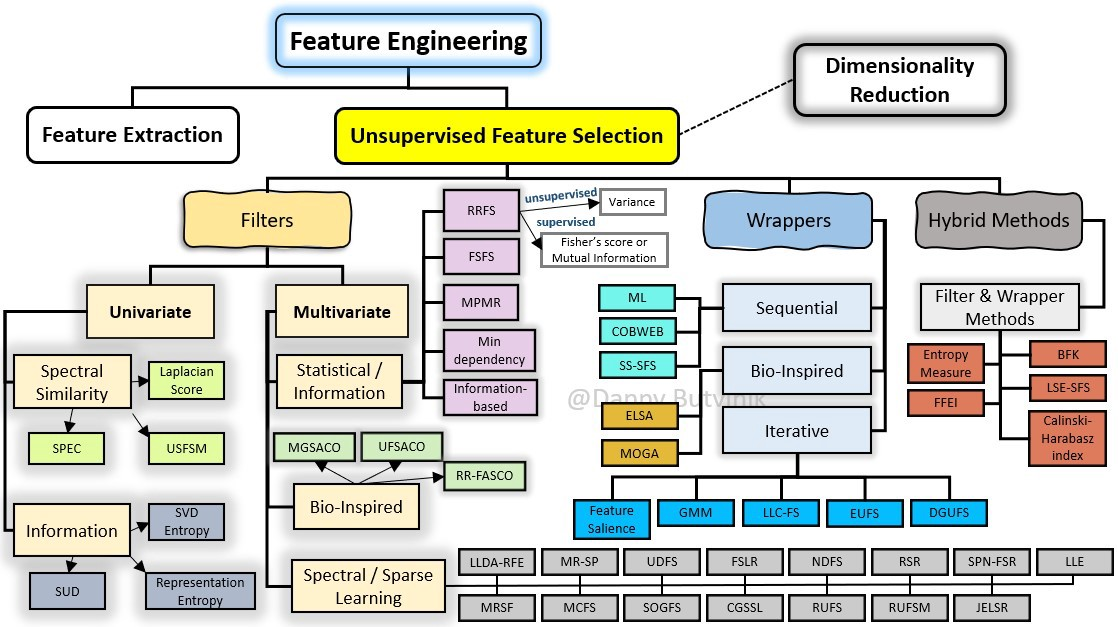scikit-learn 的Lasso实现中，更常用的其实是LassoCV(沿着正则化路径具有迭代拟合的套索（Lasso）线性模型)，它对超参数$\alpha$使用了交叉验证，来帮忙我们选择一个合适的$\alpha$。不过GridSearchCV+Lasso也能实现调参，这里就列一下LassoCV的参数、属性和方法。



### 参数

eps：路径的长度。eps=1e-3意味着alpha_min / alpha_max = 1e-3。 n_alphas:沿正则化路径的Alpha个数，默认100。 alphas：用于计算模型的alpha列表。如果为None，自动设置Alpha。 fit_intercept：是否估计截距，默认True。如果为False，则假定数据已经中心化。 tol：优化的容忍度，默认1e-4：如果更新小于tol，优化代码将检查对偶间隙的最优性，并一直持续到它小于tol为止 cv：定交叉验证拆分策略

### 属性

alpha_：交叉验证选择的惩罚量 coef_：参数向量（目标函数公式中的w）。 intercept_：目标函数中的截距。 mse_path_：每次折叠不同alpha下测试集的均方误差。 alphas_：对于每个l1_ratio，用于拟合的alpha网格。 dual_gap_：最佳alpha（alpha_）优化结束时的双重间隔。 n_iter_ int：坐标下降求解器运行的迭代次数，以达到指定容忍度的最优alpha。

### 方法

fit(X, y[, sample_weight, check_input]) 用坐标下降法拟合模型。 get_params([deep]) 获取此估计器的参数。 path(X, y, [, l1_ratio, eps, n_alphas, …]) 计算具有坐标下降的弹性网路径。 predict(X) 使用线性模型进行预测。 score(X, y[, sample_weight]) 返回预测的确定系数R ^ 2。 set_params(*params) 设置此估算器的参数。 

## 导入库

import numpy as np import pandas as pd import matplotlib.pyplot as plt from sklearn.model_selection import train_test_split from sklearn.preprocessing import StandardScaler from sklearn.linear_model import Lasso import warnings warnings.filterwarnings('ignore')

## 读取数据

url = r'F:\100-Days-Of-ML-Code\datasets\Regularization_Boston.csv' df = pd.read_csv(url)

scaler=StandardScaler() df_sc= scaler.fit_transform(df) df_sc = pd.DataFrame(df_sc, columns=df.columns) y = df_sc['price'] X = df_sc.drop('price', axis=1) # becareful inplace= False X_train, X_test, y_train, y_test = train_test_split(X, y, test_size=0.2) 

Lasso调参数，主要就是选择合适的alpha，上面提到LassoCV，GridSearchCV都可以实现，这里为了绘图我们手动实现。

 alpha_lasso = 10**np.linspace(-3,1,100) lasso = Lasso() coefs_lasso = []

for i in alpha_lasso: lasso.set_params(alpha = i) lasso.fit(X_train, y_train) coefs_lasso.append(lasso.coef_)

plt.figure(figsize=(12,10)) ax = plt.gca() ax.plot(alpha_lasso, coefs_lasso) ax.set_xscale('log') plt.axis('tight') plt.xlabel('alpha') plt.ylabel('weights: scaled coefficients') plt.title('Lasso regression coefficients Vs. alpha') plt.legend(df.drop('price',axis=1, inplace=False).columns) plt.show()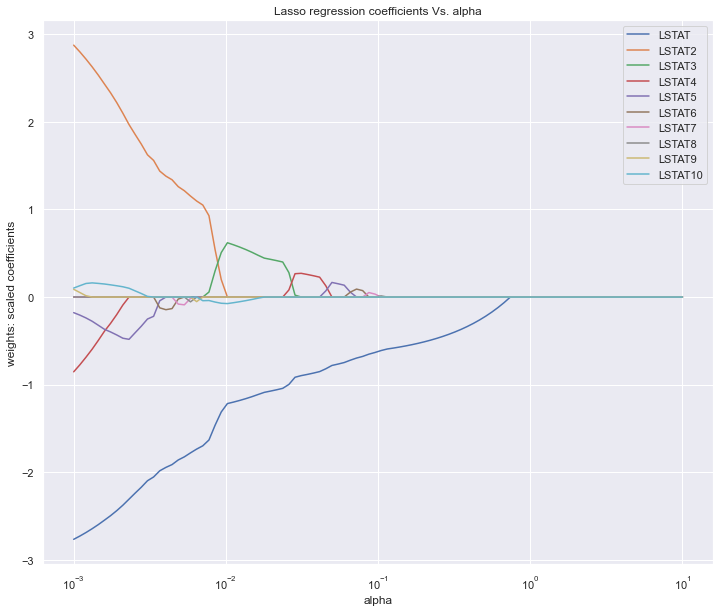图中展示的是不同的变量随着alpha惩罚后，其系数的变化，我们要保留的就是系数不为0的变量。alpha值不断增大时系数才变为0的变量在模型中越重要。

LSTAT 2.766566
LSTAT4 0.853773
LSTAT5 0.178117
LSTAT10 0.102558
LSTAT9 0.088525
LSTAT8 0.001112
dtype: float64 lasso = Lasso(alpha=10**(-2)) model_lasso = lasso.fit(X_train, y_train) coef = pd.Series(model_lasso.coef_,index=X_train.columns) print(coef[coef != 0].abs().sort_values(ascending = False))

LSTAT 1.220552
LSTAT3 0.625608
LSTAT10 0.077125
dtype: float64

a = a.sort_values('importance',ascending = False) plt.figure(figsize=(12,8)) plt.barh(a['feature'],a['importance']) plt.title('the importance features') plt.show()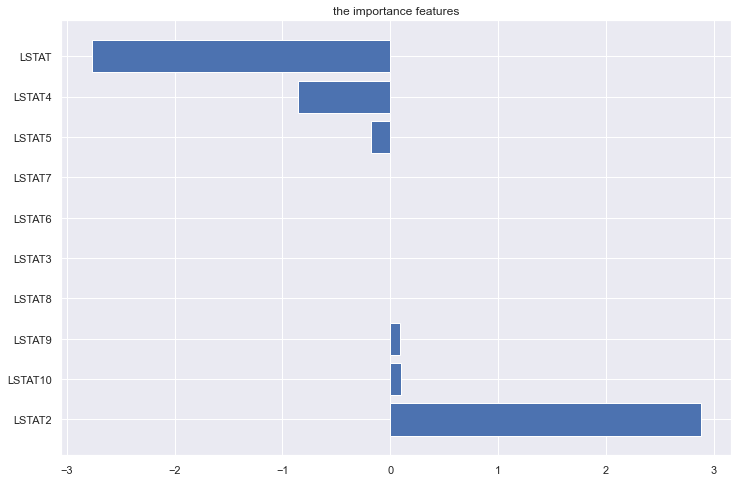## 总结

Lasso回归方法的优点是可以弥补最小二乘估计法和逐步回归局部最优估计的不足，可以很好地进行特征的选择，有效地解决各特征之间存在多重共线性的问题。

## reference

https://www.biaodianfu.com/ridge-lasso-elasticnet.html
https://machinelearningcompass.com/machine_learning_models/lasso_regression/
https://www.cnblogs.com/pinard/p/6004041.html
https://www.biaodianfu.com/ridge-lasso-elasticnet.html